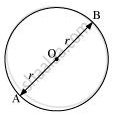# Write True or False. Give reasons for your answers. A chord of a circle, which is twice as long as its radius, is a diameter of the circle. - Mathematics

MCQ
True or False

A chord of a circle, which is twice as long as its radius, is a diameter of the circle.

• True

• False

#### Solution

True.

Let AB be a chord which is twice as long as its radius. It can be observed that in this situation, our chord will be passing through the centre of the circle. Therefore, it will be the diameter of the circle.Concept: Concept of Circle - Centre, Radius, Diameter, Arc, Sector, Chord, Segment, Semicircle, Circumference, Interior and Exterior, Concentric Circles
Is there an error in this question or solution?
Chapter 10: Circles - Exercise 10.1 [Page 171]

#### APPEARS IN

NCERT Class 9 Maths
Chapter 10 Circles
Exercise 10.1 | Q 2.4 | Page 171
Share